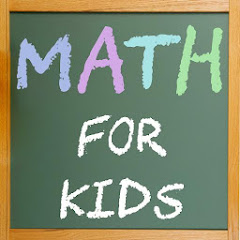10K+Everyone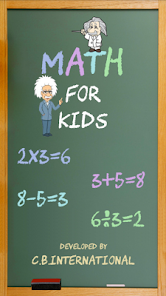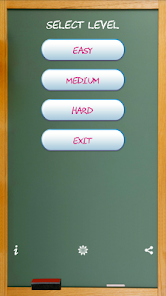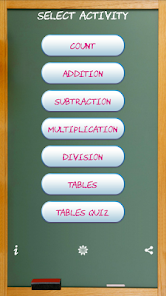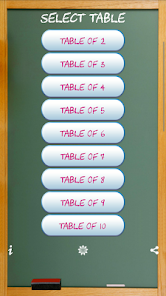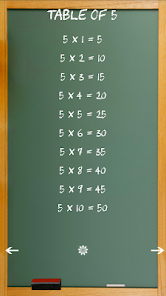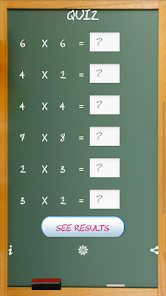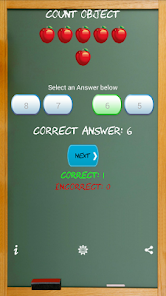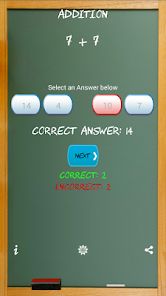Math for Kids:Basic Addition, Subtraction, Multiplication, Division and Tables

Learn Math for Kids help kids learn Addition, Subtraction, Multiplication, Division and Tables

In Math for Kids children can choose levels Easy, Medium and Hard of mathematics exercises that include Additions, Subtractions, Multiplications, Divisions and children also can learn Multiplication Tables and can take quiz for tables.

This app is an educational math Quiz for kids and a perfect math workout for kids!
★ Subtractions
★ Multiplications
★ Divisions
★ Count Objects- Counting objects from 1 to 10 for kids
★ Tables – Multiplication Tables from 1 to 20.

Easy Level:
★ Only single digit numbers are provided to kids in all the actions.
★ Multiplication Table Quiz is upto table of 8.

Medium Level:
★ Only numbers upto two digits are provided to kids in all the actions.
★ Multiplication Table Quiz is upto table of 15.

Hard Level:
★ Three digit numbers are provided to kids in all the actions.
★ Multiplication Table Quiz is upto table of 20.
Kids Math. Please take out a minute to Rate and Review our app.